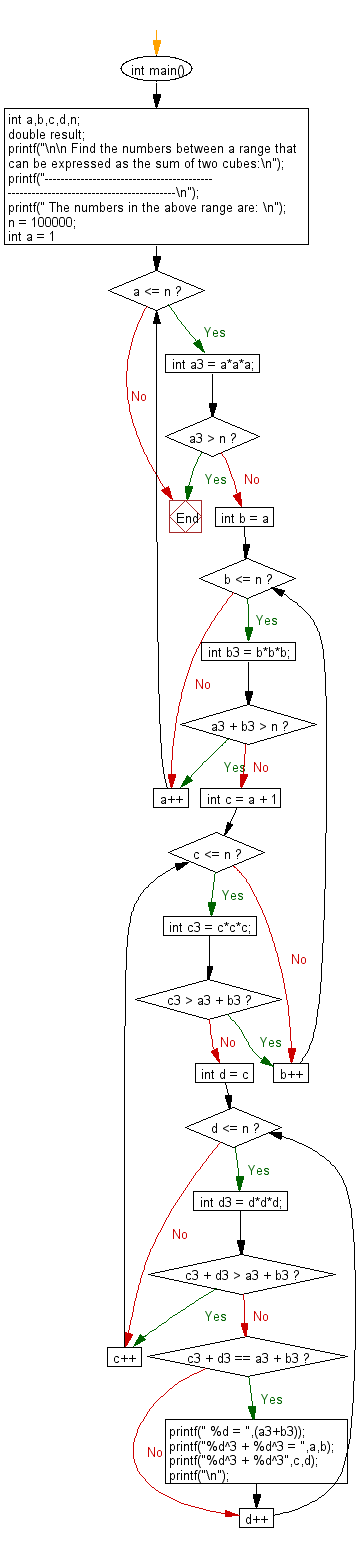﻿ C Program: Sum of two cubes between 1 and n# C Exercises: Find any number between 1 and n that can be expressed as the sum of two cubes

## C Numbers: Exercise-31 with Solution

Write a program in C to find any number between 1 and n that can be expressed as the sum of two cubes in two (or more) different ways.

Sample Solution:

C Code:

``````# include <stdio.h>
# include <stdlib.h>
# include <math.h>

int main()
{
int a,b,c,d,n;
double result;
printf("\n\n Find the numbers between a range that can be expressed as the sum of two cubes:\n");
printf("------------------------------------------------------------------------------------\n");
printf(" The numbers in the above range are: \n");
n = 100000;
for (int a = 1; a <= n; a++)
{
int a3 = a*a*a;
if (a3 > n) break;

for (int b = a; b <= n; b++)
{
int b3 = b*b*b;
if (a3 + b3 > n) break;

for (int c = a + 1; c <= n; c++)
{
int c3 = c*c*c;
if (c3 > a3 + b3) break;

for (int d = c; d <= n; d++)
{
int d3 = d*d*d;
if (c3 + d3 > a3 + b3) break;

if (c3 + d3 == a3 + b3)
{
printf(" %d = ",(a3+b3));
printf("%d^3 + %d^3 = ",a,b);
printf("%d^3 + %d^3",c,d);
printf("\n");
}
}
}
}
}
}
```
```

Sample Output:

``` The numbers in the above range are:
1729 = 1^3 + 12^3 = 9^3 + 10^3
4104 = 2^3 + 16^3 = 9^3 + 15^3
13832 = 2^3 + 24^3 = 18^3 + 20^3
39312 = 2^3 + 34^3 = 15^3 + 33^3
46683 = 3^3 + 36^3 = 27^3 + 30^3
32832 = 4^3 + 32^3 = 18^3 + 30^3
40033 = 9^3 + 34^3 = 16^3 + 33^3
20683 = 10^3 + 27^3 = 19^3 + 24^3
65728 = 12^3 + 40^3 = 31^3 + 33^3
64232 = 17^3 + 39^3 = 26^3 + 36^3
```

Pictorial Presentation:Flowchart:C Programming Code Editor:

What is the difficulty level of this exercise?

Test your Programming skills with w3resource's quiz.

﻿

## C Programming: Tips of the Day

Why do C and C++ compilers allow array lengths in function signatures when they're never enforced?

It is a quirk of the syntax for passing arrays to functions.

Actually it is not possible to pass an array in C. If you write syntax that looks like it should pass the array, what actually happens is that a pointer to the first element of the array is passed instead.

Since the pointer does not include any length information, the contents of your [] in the function formal parameter list are actually ignored.

Ref : https://bit.ly/3fhlvdH